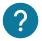# What is an integer? How to do operations with it? How to convert a number in an integer variable?

The integer variables are used to store integer numbers in your program. It corresponds to all negative and positive numbers without comma.

This is an example:

``int variable= 100;  ``

## Arithmetic Operation

With theses integers, you can do mathematical operations such as addition, subtraction or more.

Here are some examples:

``````void setup() {
Serial.begin(9600); // We initialize the serial monitor
int variable_a=13; // First variable
int variable_b=2; // Second variable
Serial.println(variable_a+variable_b); // We display the result of the addition
}

void loop() {
}``````

This is a table of all the mathematical operations that can be possible on the integers:

Operator Program
Subtraction: – int soustraction =5 – 5 // Return 0 in subtraction’s variable
Multiplication: * int multiplication = 7*2; // Return 14 in multiplication’s variable
Divison: / int division = 6/2; // Return 3 in the division’s variable
Modulo: % int modulo =7 % 5 // Return 2 in the modulo’s variable
• The maximum of two integer numbers

The maximum of two integer numbers will return the number which are the most important. We will see an example just below:

``````void setup() {
Serial.begin(9600); // We initialize the serial monitor
int variable_a=2; // We initialize the first variable Initalise la premiere variable
int variable_b=4; // We initialize the second variable
int variable_max=max(variable_a,variable_b); // The max's variable return the maximum of the two variables.
Serial.print("The maximum is:"); // We display the maximum between the two numbers
Serial.println(variable_max);
}

void loop() {
}``````

You can also compare two integer variables in a if, for or while loop, with comparison operators. You can find theses operators our course about mathematics function.### How to convert our value in an integer?

It is possible that a you need to transform a number from a float’s variable, string to an integer’s variable. To do that you will need to add (int) before your variable to transform it to integer’s variable:

``````void setup() {
Serial.begin(9600); // We initialize the serial monitor
float variable_float =23.0; // Your float's variable
int variable_integer = (int)variable_float; // To convert the variable in an integer
Serial.println(variable_integer); // We display 23
}

void loop() {
}``````

You can also directly use a float’s variable or a string’s variable and convert it to an integer:

``````void setup() {
Serial.begin(9600);
String variable ="100.56";
int variable_integer=variable.toInt(); // Transform 100.56 into the integer 100
Serial.println(variable_integer);
}

void loop() {
}``````

By converting a the value of a string’s variable into an integer, it will take the value in the ASCII table of your characters:

``int variable ="ee"; // Converted in 274``